+

# More Challenging Quadratic Factoring

##### Rating:
(0)
Author: Sophia Tutorial
##### Description:

Factor a quadratic expression in the form ax²+bx+c.

(more)
Tutorial
what's covered
1. Review of Basic Quadratic Factoring
2. Factoring when a ≠ 1
3. The Box Method

# 1. Review of Basic Quadratic Factoring

Factoring a quadratic is the opposite process of FOILing: we are taking a quadratic expression, and rewriting it as two binomials being multiplied together. With basic factoring, we are seeking to identify two integers, p and q, such that:

• The product of p and q is the constant term of the quadratic
• and the sum of p and q is the coefficient of the x-term in the quadratic

For example, the quadratic expression x2 – 5x + 6 factors to (x – 2)(x – 3), because -2 • -3 is +6, and -2 + (-3) is -5.

# 2. Factoring when a ≠ 1

Things get more complicated when the leading coefficient of the quadratic is other than 1. This is because at least one of the binomials in factored form must have a coefficient of x that is other than 1. If we start with FOIL, we can see this pattern:

(ax + p)(x+q) = ax2 + aqx + px +pq = ax2 + (aq+p)x + pq

When factoring these kinds of quadratics, we still start with the product of p and q, pq. We break down the constant term into two integers that will multiply to equal that constant term. However, when we add the numbers together to match up with the x-term coefficient, we must first multiply q by a (this is seen in the aqx term above).

Let's use this pattern to factor the quadratic: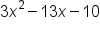These are our candidates for what p and q can be. To determine which pair of integers we will use, we must first multiply q by 3 (because that is our leading coefficient) and then add to p:Since we are looking for a sum of –13, we have identify p as 2 and q as –5 to use when factoring: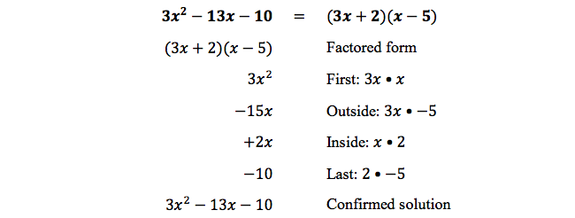# 3. The Box Method

Another method to factoring quadratics with a leading coefficient other than 1 is to use the box method. Let's use the box to factor the same quadratic as above:.

We begin by multiplying the leading coefficient of x-squared by the constant term, and identify two integers that multiply to this value. 3 times -10, so we are going to list two integers that multiply together to equal -30.Once again, we want the sum of these two integers to match the x-term coefficient, which is -13. For this reason, we will use 2 and -15. However, these two values are not use in the same way as before. We already know that (x + 2)(x – 15) does not equal 3x2–13x–10.

Instead, we use these two numbers in a 2x2 grid, along with the x-squared term and the constant term. To start, write the original x-squared term and the constant term in the corners of a 2x2 grid: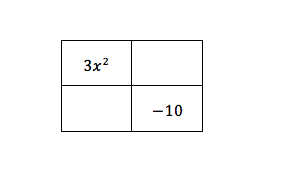Then, we use 2 and -15 (identified from earlier) as coefficients to x terms to be included in the 2x2 box: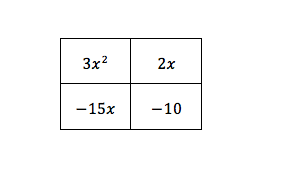hint
It does not matter which squares the terms go into. Either way, you'll get the same answer. Also note that the sum of all of the terms in the square equals our quadratic.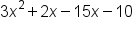is equal to, our original quadratic. If the sum doesn't add up, you've made an error somewhere.
So what do we do to this grid now? We look at the rows and columns, and factor out the greatest common factor in each row and column, and write it outside of the grid. What's left outside of the grid are the two factors to write the quadratic in factored form: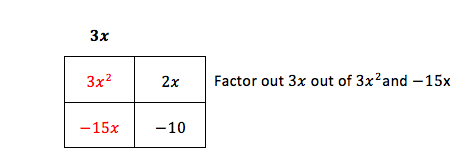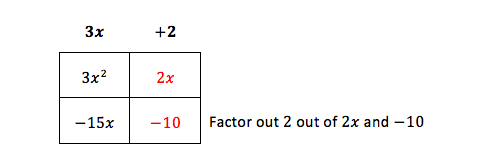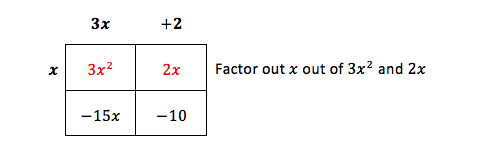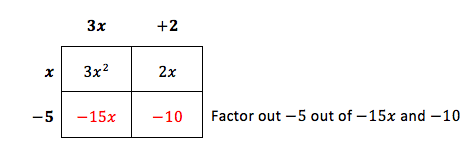What we factored outside of the box forms the binomials used in factored form: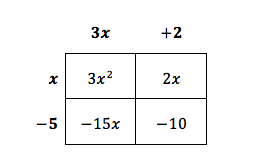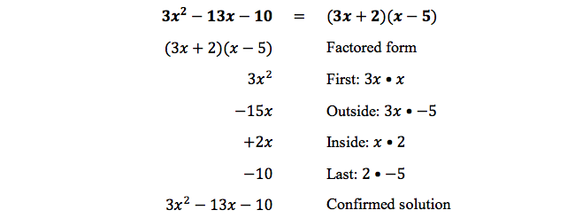summary
Factoring is the process of writing an equation from expanded form to factored form. A review of basic quadratic factoring (when the x-squared coefficient is equal to 1), involves finding two integers whose sum is the coefficient of the x term and whose product is the constant term. Factoring when a ≠ 1 involves multiplying the leading coefficient of x-squared, or a, by the constant term, and identify two integers that multiply to this value. This is also known as the box method.

Rating Header